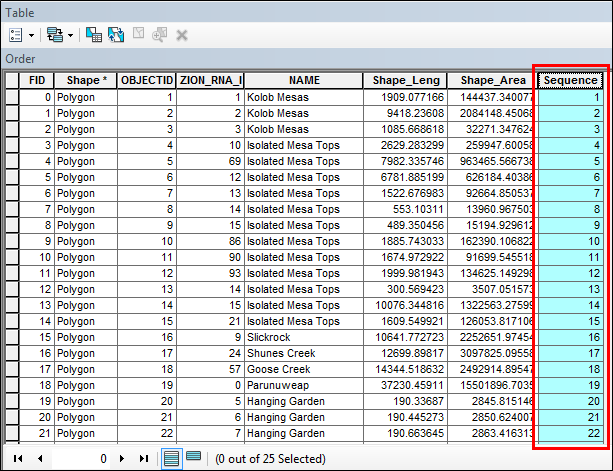HOW TO

# Create sequential numbers in a field in ArcMap using Python in the Field Calculator

Last Published: April 9, 2023

## Summary

Instructions provided describe how to create sequential numbers in a field in ArcMap using Python in the Field Calculator.

## Procedure

```Note:
The code in this article generates sequential numbers for unsorted data based on the OID or FID order. If the data is sorted on a field, the generated numbers are not sequential.```
1. Create a new short integer field. Refer to ArcMap: Adding fields for instructions.
2. Right-click the new field and select Field Calculator.
3. Set the Parser to Python.
4. Check the check box for Show Codeblock.
5. Paste the following into the Pre-Logic Script Code:
```rec=0
def autoIncrement():
global rec
pStart = 1
pInterval = 1
if (rec == 0):
rec = pStart
else:
rec += pInterval
return rec```
1. Paste the following code in the smaller box below the Pre-Logic Script Code:
`autoIncrement()`
1. Click OK. The following image is an example of a field with sequential numbers populated using the Python code:Article ID:000011137

Software:
• ArcMap

Get help from ArcGIS experts

Contact technical support